Next: LC Circuits Up: Simple Harmonic Oscillation Previous: Mass on a Spring

# Simple Harmonic Oscillator Equation

Suppose that a physical system possessing a single degree of freedom--that is, a system whose instantaneous state at time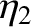is fully described by a single dependent variable,--obeys the following time evolution equation [cf., Equation (2)],(17)

where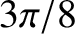is a constant. As we have seen, this differential equation is called the simple harmonic oscillator equation, and has the solution(18)

whereandare constants. Moreover, this solution describes a type of oscillation characterized by a constant amplitude,, and a constant angular frequency,. The phase angle,, determines the times at which the oscillation attains its maximum value. The frequency of the oscillation (in hertz) is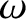, and the period is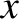. The frequency and period of the oscillation are both determined by the constant, which appears in the simple harmonic oscillator equation, whereas the amplitude,, and phase angle,, are determined by the initial conditions. [See Equations (10)-(13).] In fact,andare the two arbitrary constants of integration of the second-order ordinary differential equation (17). Recall, from standard differential equation theory (Riley 1974), that the most general solution of anth-order ordinary differential equation (i.e., an equation involving a single independent variable, and a single dependent variable, in which the highest derivative of the dependent with respect to the independent variable isth-order, and the lowest zeroth-order) involvesarbitrary constants of integration. (Essentially, this is because we have to integrate the equationtimes with respect to the independent variable to reduce it to zeroth-order, and so obtain the solution. Furthermore, each integration introduces an arbitrary constant. For example, the integral of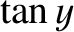, whereis a known constant, is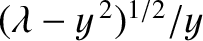, where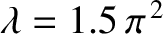is an arbitrary constant.)

Multiplying Equation (17) by, we obtain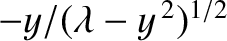(19)

However, this can also be written(20)

or(21)

where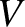(22)

According to Equation (21),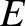is a conserved quantity. In other words, it does not vary with time. This quantity is generally proportional to the overall energy of the system. For instance,would be the energy divided by the mass in the mass-spring system discussed in Section 2.1. The quantityis either zero or positive, because neither of the terms on the right-hand side of Equation (22) can be negative.

Let us search for an equilibrium state. Such a state is characterized by, so that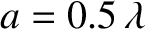. It follows from Equation (17) that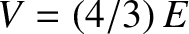, and from Equation (22) that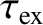. We conclude that the system can only remain permanently at rest when. Conversely, the system can never permanently come to rest when, and must, therefore, keep moving for ever. Because the equilibrium state is characterized by, we deduce that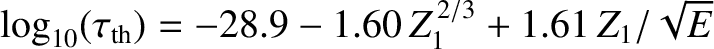represents a kind of displacement'' of the system from this state. It is also apparent, from Equation (22), thatattains it maximum value when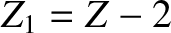. In fact,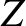(23)

where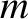is the amplitude of the oscillation. Likewise,attains its maximum value,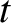(24)

when.

The simple harmonic oscillation specified by Equation (18) can also be written in the form(25)

whereand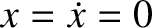. Here, we have employed the trigonometric identity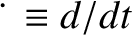. (See Appendix B.) Alternatively, Equation (18) can be written(26)

where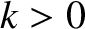, and use has been made of the trigonometric identity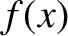. (See Appendix B). It follows that there are many different ways of representing a simple harmonic oscillation, but they all involve linear combinations of sine and cosine functions whose arguments take the form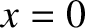, whereis some constant. However, irrespective of its form, a general solution to the simple harmonic oscillator equation must always contain two arbitrary constants. For example,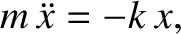andin Equation (25), orand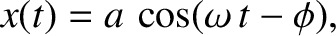in Equation (26).

The simple harmonic oscillator equation, (17), is a linear differential equation, which means that ifis a solution then so is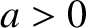, whereis an arbitrary constant. This can be verified by multiplying the equation by, and then making use of the fact that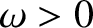. Linear differential equations have the very important and useful property that their solutions are superposable. This means that ifis a solution to Equation (17), so that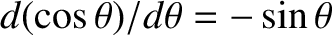(27)

and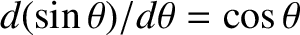is a different solution, so that(28)

then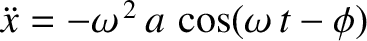is also a solution. This can be verified by adding the previous two equations, and making use of the fact that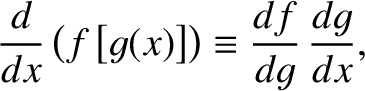. Furthermore, it can be demonstrated that any linear combination of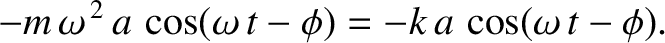and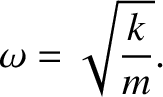, such as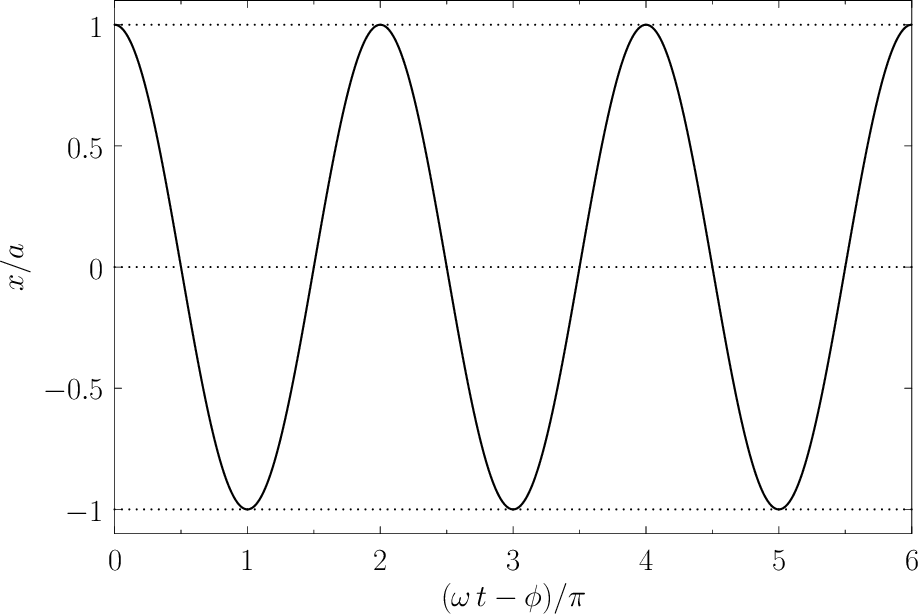, whereandare constants, is also a solution. It is very helpful to know this fact. For instance, the special solution to the simple harmonic oscillator equation, (17), with the simple initial conditions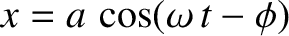and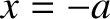can be shown to be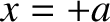(29)

Likewise, the special solution with the simple initial conditions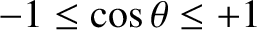and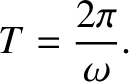is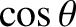(30)

Thus, because the solutions to the simple harmonic oscillator equation are superposable, the solution with the general initial conditionsand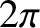becomes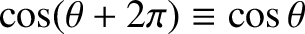(31)

or(32)Next: LC Circuits Up: Simple Harmonic Oscillation Previous: Mass on a Spring
Richard Fitzpatrick 2013-04-08